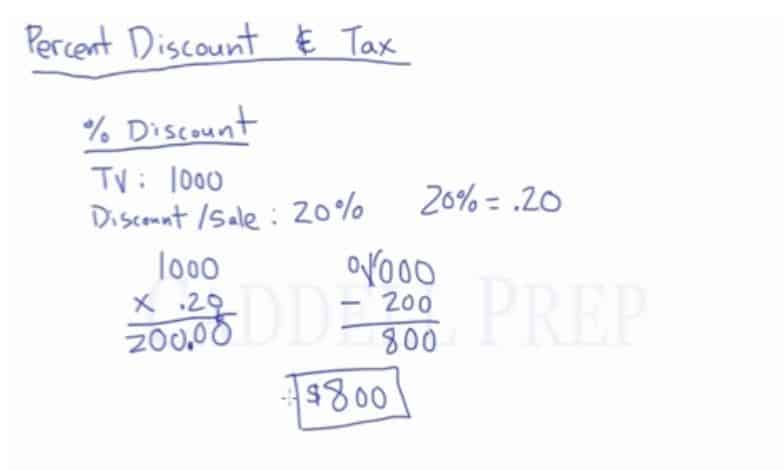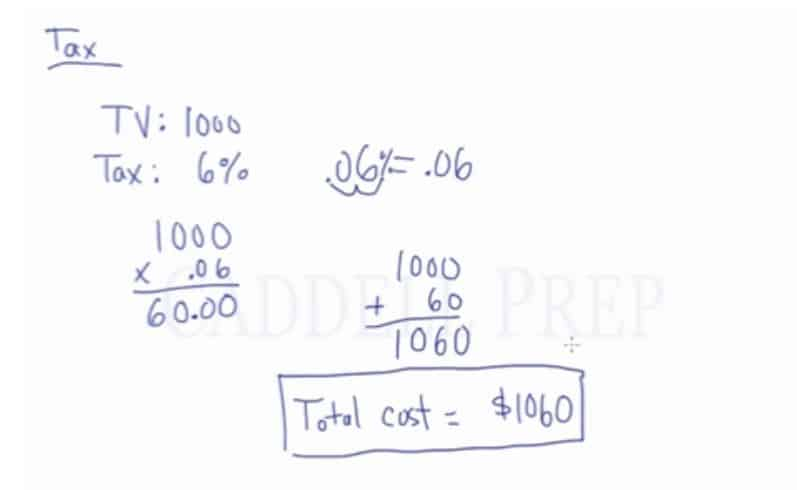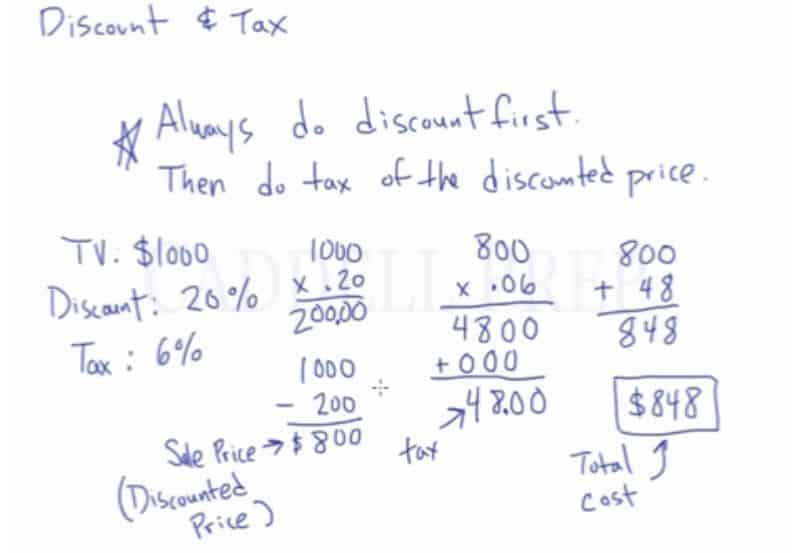## Examples of Discount & Tax

### Example 1

Megan bought a bracelet for$\120$. She received a$15\%$ discount, and she paid a sales tax of$6\%$. How much did she pay for the bracelet?

The rule in solving discount and tax is “Always do discount first then do tax of the discounted price”

First, let’s solve for the discount$120 \times 0.15=18$

Then, subtract to get the discounted price$120-18= 102$

The$\102$ is the sale price/discounted price.

Now, let’s get the tax$102 \times 0.06= 6.12$

The tax is$\6.12$

To get the final price, we have to add the discounted price to the tax.$102 + 6.12 = 108.12$

Our total cost now is$\108.12$

### Example 2

Eric bought a laptop for$\350$. He was given$5\%$ discount. The sales tax rate is$8\%$. What is the sale price including tax?

The rule in solving discount and tax is “Always do discount first then do tax of the discounted price”

First, let’s solve for the discount$350 \times 0.05=17.5$

Then, subtract to get the discounted price$350-17.5= 332.5$

The$\332.5$ is the sale price/discounted price.

Now, let’s get the tax$332.5 \times 0.08= 26.6$

The tax is$\26.6$

To get the final price, we have to add the discounted price to the tax.$332.5 + 26.6 = 359.1$

Our total cost now is$\359.1$

## Video-Lesson Transcript

In this lesson, we will discuss percent discount and tax.

### Percent Discount

First, let’s discuss percent discount.

Percent discount is how much you’re going to receive off of a price.

For example, a TV had an original price of$\1,000$ which was put on sale at$20\%$.

Some are confused with$20\%$. This is not a value, it’s a ratio or a fraction.

So here we should find out what is$20\%$ of$\1,000$ then subtract that.

Let’s compute it$1,000 \times 0.20$

Just a side note:$20\% = 0.20$

So,$1,000 \times 0.20 = 200.00$$200$ is the discount. This is what we should take off of the price.

In the example, since the TV was originally priced at$\1,000$, we should subtract the discount of$\200$.$1,000 - 200 = 800$

The discounted price of the TV is$\800$.Again, the$20\%$ is not the actual price. We should find out what is$20\%$ of$\1,000$ then subtract it from the original price of$\1,000$ to get the discounted price.

### Tax

Now, let’s move on to tax.

Tax is added to a price.

For example, a different TV is priced at$\1,000$ with a tax of$6\%$.

So we have to find out what is$6\%$ of$\1,000$. Then add it to get the final price.

Side note:$6\% = 0.06$

Let’s compute:$1,000 \times 0.06 = 60.00$

So now the final price is computed as the original price of the TV plus the tax.$1,000 + 60 = 1,060$

The total cost is$\1,060$.So for tax, find the amount then add it on the original price.

### Discount and Tax

Let’s have more complicated problems involving discount and tax.

A very important rule in discount and tax is to:
Always do discount first then do tax of the discounted price.

For example, we have a TV priced at$\1,000$, discounted for$20\%$ and should be taxed at$6\%$.

To compute, let’s get the discount first.$1,000 \times 0.20 = 200.00$

Then subtract to get the discounted price$1,000 - 200 = 800$

The$\800$ is the sale price/discounted price.

Then let’s get the tax$800 \times 0.06 = 48$

The tax is$\48$

So to get the final price we have to add the discounted price to the tax.$800 + 48 = 848$

Our total cost now is$\848$Let’s have a quick recap.

We have a TV priced at$\1,000$, discounted for$20\%$ and should be taxed at$6\%$.

We got the discount of$\200$ which was subtracted to the original price of$\1,000$, the answer is$\800$.$\800$ is the price we’re paying for the TV. The same price we’re going to use to get the tax.

The tax was computed by$\800$ multiplied by the tax$6\%$ where we got the tax of$\48$.

So the total cost is computed by$\800$ for the TV plus the tax of$\48$.

So the total cost of the TV is$\848$.

After you finish this lesson, view all of our Pre-Algebra lessons and practice problems.